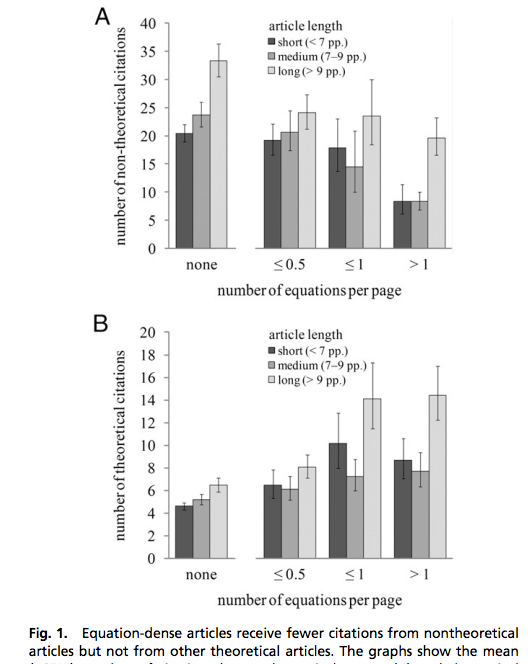## Tuesday, June 26, 2012

### Why non-theoreticians don’t cite your paper

T.W. Fawcett and A. D. Higginson. 2012. Heavy use of equations impedes communication among biologists. PNAS.

The more equations a paper has, the less it will be cited by other biologists. This should come as a surprise to few people, but if it does, Fig.1 from Fawcett and Higginson (2012) makes this pretty clear. Papers with many equations per page are cited less often by non-theoretical papers (A). In fact, citations by non-theoretical papers decrease by 35% for each additional equation per page. This is not true of theoretical papers, which happily cite other equation-filled theoretical works (B). It’s an interesting conundrum: theory unifies empirical observations and generates predictions, but theory uses equations. And papers with equations have less impact.

The authors make suggestions for both sides of this divide. All biologists should have adequate mathematical training so that equations are not necessarily considered daunting or confusing. Theoreticians should strive to communicate their works in accessible ways (something Steve Ellner covered nicely in the aptly named “How to write a theoretical ecology paper that people will cite”). The authors also suggest increased placement of equations in appendices, where they do not decrease citation rates. (However, if equations don’t decrease citation rates when in the appendix, you wonder if this is because equations are easier to ignore there). The surprising thing about this  bias is that I don’t think it exists as much in the other direction. Theoretical papers generally do cite empirical works. Reviewers frequently require that model assumptions be justified based on empirical knowledge. A balance between theory and empiricism seems important for ecology, and while this paper doesn't tell us anything surprising, it makes it quite clear that there is a problem.From Fawcett and Higginson 2012.